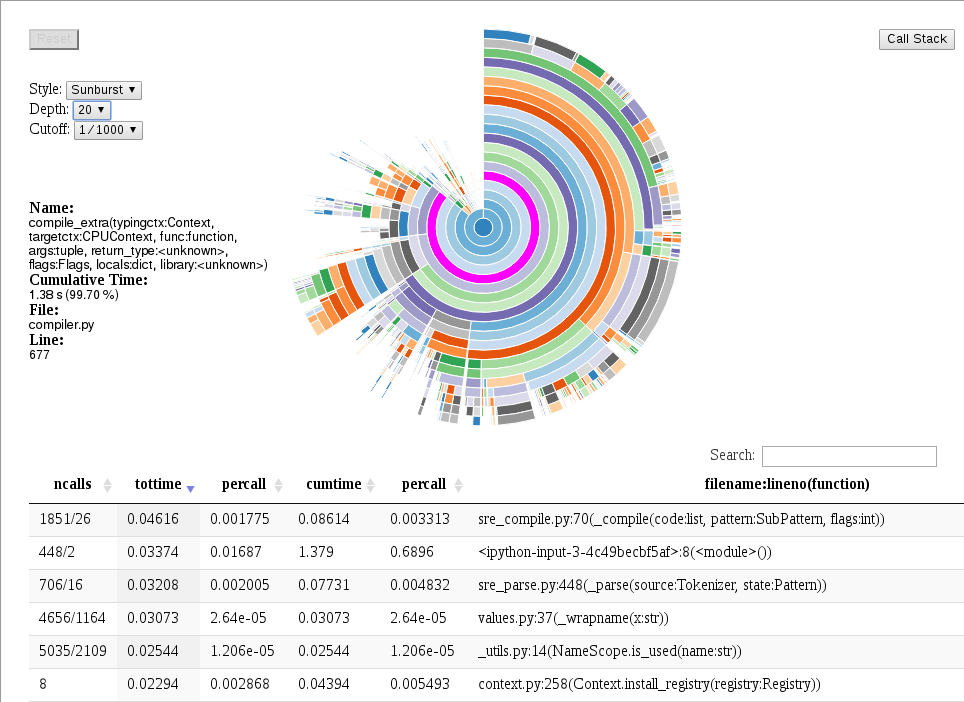# 分析¶

Python 标准库包括代码分析功能。当活动时，函数调用和花费在它们上的时间被记录。

`accelerate.profiler`模块还通过记录函数的签名来扩展此功能，这很有用，因为通常精确的控制流以及函数性能取决于参数类型。对于 NumPy 数组类型，这不仅包括`dtype`属性，还包括数组的形状。

## 分析示例¶

```from accelerate import profiler
import numpy as np

def dot(a, b):
sum=0
for i in range(len(a)):
sum += a[i]*b[i]
return sum

a = np.arange(16, dtype=np.float32)
b = np.arange(16, dtype=np.float32)

p = profiler.Profile(signatures=False)
p.enable()
dot(a, b)
p.disable()
p.print_stats()
```

```      3 function calls in 0.000 seconds

Ordered by: standard name

ncalls  tottime  percall  cumtime  percall filename:lineno(function)
1    0.000    0.000    0.000    0.000 builtins.len
1    0.000    0.000    0.000    0.000 dot.py:7(dot)
1    0.000    0.000    0.000    0.000 {method 'disable' of 'prof.Profiler' objects}
```

```      3 function calls (2 primitive calls) in 0.000 seconds

Ordered by: standard name

ncalls  tottime  percall  cumtime  percall filename:lineno(function)
1    0.000    0.000    0.000    0.000 dot.py:1(disable())
2/1    0.000    0.000    0.000    0.000 dot.py:7(dot(a:ndarray(dtype=float32, shape=(16,)), b:ndarray(dtype=float32, shape=(16,))))
```

```In : profiler.plot(p)
```## Accelerate.profiler API ¶

`accelerate.profiler.``Profile`( custom_timer=None , time_unit=None , subcalls=True , builtins =True , signatures=True )

`clear`( )

`disable`( )

`enable`( subcalls=True , builtins= True )

`getstats`( ) → profiler_entry 对象列表

call 属性要么是 None 要么是一个 profiler_subentry 对象列表：

`print_stats`(排序=-1 )

`accelerate.profiler.``plot`(探查器)

profiler 中生成当前配置文件统计信息的可视化。目前这仅支持交互式笔记本